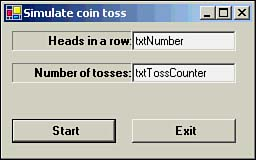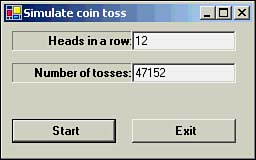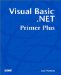# Do While LoopsFlavor One

### Do While Loops ”Flavor One

Several flavors of Do - While loops are available to you. The major difference between them is where the relational test is performed. The first version we will explore has the syntax form:

`  Do While   Expression1   DoWhileStatementBlock   Loop  `

Expression1 is a Boolean expression that evaluates to either logic True or False. If Expression1 is logic True, the statements in the Do - While statement block (that is, DoWhileStatementBlock ) are executed. When the Loop keyword is reached, control is directed back to the Do - While test on Expression1 . As long as Expression1 is logic True, the loop statements continue to be executed. When Expression1 becomes logic False, control is sent to the first statement following Loop .

For example, let's write a program that simulates tossing a coin. Let's further assume that we want to see how many coin tosses it takes before we get a certain number of heads in a row. Start a new project named CoinTosses and add the objects shown in Figure 13.2.

##### Figure 13.2. The placement of objects for the CoinTosses project.The first text box, named txtNumber , is the number of heads we want in a row. Text box txtTossCounter shows how many tosses were made before we got the desired number of heads. Consider Listing 13.4 for the btnStart Click event.

##### Listing 13.4 Code for the btnStart Click Event
` Private Sub btnStart_Click(ByVal sender As System.Object, ByVal e As _               System.EventArgs) Handles btnStart.Click  Dim Tosses, Heads, ThisToss, Target As Integer  Heads = 0  Tosses = 0  If txtNumber.Text <> "" Then   ' Make sure they entered a number   Target = CInt(txtNumber.Text)  Else   MessageBox.Show("You need to enter a number.")   Exit Sub  End If  Do While Heads <> Target   ThisToss = TossACoin()  ' Function returns 1 for head   If ThisToss = 1 Then    Heads += 1       ' It was a Heads, increment the count   Else    Heads = 0        ' Tails...start over   End If   Tosses += 1        ' The toss counter  Loop  txtTossCounter.Text = CStr(Tosses) End Sub `

Notice how we check to make sure that the user entered a number into the txtNumber text box. If the user didn't enter a number, an error message is displayed and we leave the sub-routine.

We've written a function named TossACoin() that returns a 1 if a heads was tossed, or if a tails was the result. When we enter the Do - While loop, Heads is , which means the relational test that Heads is not equal to Target is logic True. This causes control to enter the Do - While statement block. The code for the TossACoin() is presented in Listing 13.5.

##### Listing 13.5 Function to Simulate Tossing a Coin
` Private Function TossACoin() As Integer  ' Purpose: To simulate tossing a coin. The function  '      return 1 for a Head, and 0 for a tail.  '  ' Parameters  '  ' Return value  '  integer    1 for Head, 0 for tails  '  TossACoin = Int(2 * Rnd()) End Function `

A call to the Randomize() function seeds the random number generator so that a nonrepeatable series of pseudo-random numbers is produced. The function needs to be called only once before Rnd() is used, so we placed the call in the Form_Load event. (The Randomize() function uses the system timer for this purpose.) The call to Rnd() returns a value between 0 and 1 as a Single data type. When you want a value to fall between two values, you use the formula:

` Int((upperlimit - lowerlimit + 1) * Rnd() + lowerlimit) `

Because we want either a 1 or a 0, our formula becomes

` Int((1 - 0 + 1) * Rnd() + 0)  Int(2 * Rnd()) `

which is the statement we used in Listing 13.4.

Programmer's TipIf you call Rnd() with a negative number as an argument, such as Rnd(-1) , the function returns a repeatable sequence of random numbers. That is, you'll get the same series of random numbers each time. This can be useful when debugging a program. Obviously, if you're seeding Rnd() this way, there's no reason to execute a call to Randomize() .

I'll assume that by now you're comfortable adding the code for the Exit button. Figure 13.3 shows a sample run.

##### Figure 13.3. Sample run of the CoinTosses project.As you can see from Figure 13.3, it took 47,152 tosses before we got 12 heads in a row. If you care to repeat the experiment, I've seen as few as 14 tosses give 12 heads in a row! You might want to put a breakpoint on the Do - While statement and single-step the program so that you can see how the loop works.Visual Basic .NET Primer Plus
ISBN: 0672324857
EAN: 2147483647
Year: 2003
Pages: 238
Authors: Jack Purdum

Similar book on Amazon